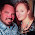## Thursday, January 16, 2014

### 33 Watch | The 50 States of Freemasonry from Delaware to Hawaii

If you look through the history books these are the confirmed Freemasons who helped found the United States of America.
1. George Washington was a Freemason
2. Benjamin Franklin was a Freemason
3. James Monroe was a Freemason
4. John Jay was a Freemason
5. John Hancock was a Freemason
6. Paul Revere was a Freemason
7. John Paul Jones was a Freemason
8. James Otis was a Freemason
9. Between 9-13 signers of the Constitution were Freemasons depending on one's source
10. At least nine signers of the Declaration of Independence were Freemasons
11. At least 33 of 74 generals in the Colonial Army were Freemasons
In Scottish Rite Freemasonry, which is what the founders practiced, the highest degree is thirty-three.  In our nation's monuments, state names, and important dates, numbers such as 555 and 33 are coded within for their spiritual purposes and superstitious energies.

The Washington Monument is 555 feet tall.  At the time of its construction it was the world's tallest structure and is still the world's tallest obelisk.  Interestingly enough the number 555 is also secretly coded into 5 of the Nation's 13 Original Colonies.  Let us examine.
1.  DELAWARE 4+5+3+1+5+1+9+5 = 33 = 6
2.  PENNSYLVANIA = 7+5+5+5+1+7+3+4+1+5+9+1 = 53 = 8 <<<
3.  NEW JERSEY = 5+5+5 1+5+9+1+5+7 = 15+28 = 43 = 7 <<<
4.  GEORGIA = 7+5+6+9+7+9+1 = 44 = 8
5.  CONNECTICUT = 3+6+5+5+5+3+2+9+3+3+2 = 46 = 10 = 1 <<<
6.  MASSACHUSETTS = 4+1+1+1+1+3+8+3+1+5+2+2+1 = 33 = 6
7.  MARYLAND = 4+1+9+7+3+1+5+4 = 34 = 7
8.  SOUTH CAROLINA = 1+6+3+2+8 3+1+9+6+3+9+5+1 = 20+37 = 57 = 12 = 3
9.  NEW HAMPSHIRE = 5+5+5 8+1+4+7+1+8+9+9+5 = 15+52 = 67 = 13 = 4 <<<
10.  VIRGINA = 4+9+9+7+9+5+9+1 = 53 = 8
11.  NEW YORK = 5+5+5 7+6+9+2 = 15+24 = 39 = 12 = 3 <<<
12.  NORTH CAROLINA = 5+6+9+2+8 3+1+9+6+3+9+5+1 = 30+37 = 67 = 13 = 4
13.  RHODE ISLAND = (9+8+6+4+5) + (9+1+3+1+5+4) = 32+23 = 55 = 10 = 1
• Delaware was established December 7, 1787 = 1+2+7+1+7+8+7 = 33
• Rhode Island established May 29, 1790 = 33
• Hawaii is the fiftieth and final state
• HAWAII = 8+1+5+1+9+9 = 33
• Hawaii was established August 21, 1959 = 8/21/1959
• 8/21 = 8+2+1 = 11
• 1959 = 1+9+5+9 = 24 = 6
• 6 x 11 = 66 (Keep the number 66 in mind)
It is the second state Pennsylvania, third state New Jersey, fifth state Connecticut, ninth state New Hampshire and eleventh state New York that have secretly coded in 555.  This isn't a coincidence, instead it is entirely meaningful.  The number 555 symbolizes the creation of something very big, which is exactly what the United States has gone on to become, through genocide, war, expansion and militaristic advancement on a level never before seen.

Two of the states, Delaware the first, and Massachusetts the sixth, sum to 33 each.  33+33 = 66, which is what the sum is of all 13 colonies when you break them down to a single digit (where 33 = 3+3 = 6).  If you sum all of the states we get a special number, 66.  66 is special because it also represents creation, due to the fact that the word woman is equal to 66.  Of course women are the creators, so this naturally makes sense.  66 is also the sum of every number added one through eleven, and the numerology of 66 is 6+6 = 12, the number that follows eleven.  For this reason, the numbers one through twelve represent completeness, because the streak is broken if you add the number 13.  In other words, 13 is a special number to, because it is the sequence ender.  When we think of 13, of course we think of the 13 original colonies and stripes on the flag.  The mathematical proof of 66 is as follows:

The 13 Colonies Combined:
1. Delaware = 6 (33)
2. Pennsylvania = 8 (555 within)
3. New Jersey = 7 (555 within)
4. Georgia = 8 (44)
5. Connecticut = 1 (555 within)
6. Massachusetts = 6 (33)
7. Maryland = 7
8. South Carolina = 3
9. New Hampshire = 4 (555 within)
10. Virginia = 8
11. New York = 3 (555 within)
12. North Carolina  = 4
13. Rhode Island = 1
14. Total = 66 = Woman = Associated with birth and creation, just like a new nation.
• WOMAN = 23+15+13+1+14 = 66
• 66 = 6+6 = 12 | 12 Represents Complete
• The first highway built across the country was Route 66

If you've ever wondered how 555 was chosen for the fake movie and television show dialing number, now you no longer have to wonder.  The number is a subconscious reminder, we live in a new nation, found in a new world, bringing about a new world order, whether we like it or not.  It is plastered everywhere from our monuments, to our state names, to important dates.  Further, as a society, we crave NEW.

New = 555New is big business.  New keeps our economy moving.  New keeps us busy.  New keeps us distracted.  New is what we worship.  555-311-1119.

1.